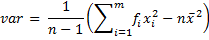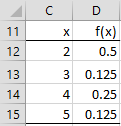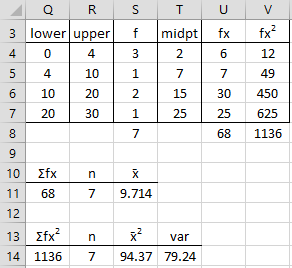# Frequency Tables

Often data is presented in the form of a frequency table. For example, the data in range A4:A11 of Figure 1 can be expressed by the frequency table in range C4:D7.Figure 1 – Frequency Table

The table in Figure 1 shows that the data element 2 occurs 4 times, the element 4 occurs 2 times and the element 3 and 5 occur 1 time.

When data is provided in the form of a frequency table, the calculation of the mean and standard deviation cannot be performed directly using the usual AVERAGE and STDEV Excel functions. In fact for sample data  {x1, …, xm} with corresponding frequency counts of f1, …, fm respectively and sample size n = f1 + f2 + … + fm, then the sample mean is (see Measures of Central Tendency):This can be calculated in Excel as

=SUMPRODUCT(R1, R2) / SUM(R2)

where R1 is a range containing the data elements {x1, …, xm}  and R2 is a range containing  {f1, …, fm}.

In a similar way, the sample variance can be calculated aswhich can be calculated in Excel as

=SUMPRODUCT((R1-R3)^2, R2)/(SUM(R2)-1)

where R1 and R2 are as above and R3 contains the sample mean (as described above). Using Property 1 of Measures of Variability, we also have the following alternative approach to calculating the variance:which can be calculated in Excel by the formula

=(SUMPRODUCT(R1^2,R2)-SUM(R2)*R3^2)/(SUM(R2)-1)

Using these formulas we can calculate the mean and variance of sample data expressed in the form of a frequency table. We demonstrate this in the following example.

Example 1: Calculate the mean and variance of the sample data from the frequency table in Figure 1.Figure 2 – Calculation of mean and variance from frequency table

The required calculation is displayed in Figure 2. Here cell F11 contains the formula =D11/E11, which calculates the mean, and cell G14 contains the formula =(D14-E14*F14)/(E14-1), which calculates the variance. The results are the same as calculating the mean and variance by applying Excel’s AVERAGE and VAR.S functions to the data set {2, 2, 2, 2, 3, 4, 4, 5}.

Note too that a frequency table is closely linked to a frequency function, as defined in Definition 1 of Discrete Distributions. E.g. since there are 8 elements in the data set in Figure 2, we see that the frequency function for the random variable x is as in Figure 3 where each frequency value is divided by 8:Figure 3 – Frequency function corresponding to frequency table

Often frequency tables are used with a range of data values, i.e. with intervals for the x values. In this case the midpoint of each interval is assigned the value xi.

Example 2: Calculate the mean and variance for the data in the frequency table in Figure 4.Figure 4 – Calculations for a frequency table with intervals

The first interval in Figure 4 is 0 < x ≤ 4, the second 4 < x ≤ 10, etc. The calculation of the mean and variance is as in Figure 2, except that now the midpoints are used as the x values.

Observation: Sometimes the first and/or last interval is unbounded: e.g. if the last interval in Figure 4 is replaced by “over 20”. In this case it isn’t possible to establish a midpoint, and so all you can do is make your best estimate of a suitable representative value for that interval.

Excel Function: When you have a lot of data, it is convenient to put the data in bins, usually of equal size, and then create a graph of the number of data elements in each bin. Excel provides the FREQUENCY(R1, R2) array function for doing this, where R1 = the input array and R2 = the bin array.

To use the FREQUENCY array function, enter the data into the worksheet and then enter a bin array. The bin array defines the intervals that make up the bins. E.g., if the bin array = 10, 20, 30, then there are 4 bins, namely data with values x ≤ 10, data with values x where 10 < x ≤ 20, data with values x where 20 < x ≤ 30, and finally data with values x > 30. The FREQUENCY function simply returns an array consisting of the number of data elements in each of the bins.

Example 3: Create a frequency table for the 22 data elements in the range A4:B14 of Figure 5 based on the bin array D4:D7 (the text “over 20” in cell D8 is not part of the bin array).Figure 5 – Example of the FREQUENCY function

To produce the output, highlight the range E4:E8 (i.e. a column range with one more cell than the number of bins) and enter the formula

=FREQUENCY(A4:B11,D4:D7)

Since this is an array formula, you must press Ctrl-Shft-Enter. Excel now inserts frequency values in the highlighted range E4:E8. Here E4 contains the number of data elements in the input range with value in the first bin (i.e. data elements whose value is ≤ 20). Similarly, E5 contains the number of data elements in the input range with value in the second bin (i.e. data elements whose value is > 20 and ≤ 40). The final output cell (E8) contains the number of data elements in the input range with value > the value of the final bin (i.e. > 80 for this example).

Observation: As described in Discrete Probability Distributions, the Real Statistics Resource Pack provides the FREQTABLE function. This function can also be used to create a frequency table with bins where the bins are equally spaced.

Real Statistics Function: The Real Statistics Resource Pack supplies the following supplemental array function to create a frequency table

FREQTABLE(R1, bsizebmax) = an array function which produces the frequency table for the data in range R1, assuming equally sized bins of size bsize where bmax is the maximum bin size value

To use the function you must highlight an array with 3 columns and at least k rows where k = (bmax – MIN(R1)) / bsize + 1. You can highlight more rows than you need; any extra rows will take value #N/A.

The bmax argument is optional. If omitted then it defaults to bmax = MAX(R1). When bmax is not omitted then you should make sure that bmax ≥ MAX(R1): otherwise some data will be lost.

The bsize argument is also optional. If omitted then instead of creating a frequency table as described above, a table with a bin for each value in R1 is used. The bmax argument is ignored. This produces a table similar to that described in Example 3 of Discrete Distributions.

Example 4: Create a frequency table for the 22 data elements in the range A4:B14 of Figure 5 based on bins of size 15.

The desired frequency table can be produced using the array formula

=FREQTABLE(A4:B14,15)

as shown in range M4:O11 of Figure 6.Figure 6 – FREQTABLE function with bin size 15

The headings are not outputted by the function but have been added manually. Note that two extra rows have been highlighted and so they are filled with #N/A.

Observation: You can also use the Histogram with Normal Curve Overlay data analysis tool for creating frequency tables. See Histograms for an example of how to use this data analysis tool. Also see Frequency Table Conversion for how to calculate the descriptive statistics for the data described by a frequency table.

Excel formula for Beginners – How to Count unique numeric values in a range in Excel

# Personal Career & Learning Guide for Data Analyst, Data Engineer and Data Scientist

## Applied Machine Learning & Data Science Projects and Coding Recipes for Beginners

A list of FREE programming examples together with eTutorials & eBooks @ SETScholars

# Projects and Coding Recipes, eTutorials and eBooks: The best All-in-One resources for Data Analyst, Data Scientist, Machine Learning Engineer and Software Developer

Topics included: Classification, Clustering, Regression, Forecasting, Algorithms, Data Structures, Data Analytics & Data Science, Deep Learning, Machine Learning, Programming Languages and Software Tools & Packages.
(Discount is valid for limited time only)`Disclaimer: The information and code presented within this recipe/tutorial is only for educational and coaching purposes for beginners and developers. Anyone can practice and apply the recipe/tutorial presented here, but the reader is taking full responsibility for his/her actions. The author (content curator) of this recipe (code / program) has made every effort to ensure the accuracy of the information was correct at time of publication. The author (content curator) does not assume and hereby disclaims any liability to any party for any loss, damage, or disruption caused by errors or omissions, whether such errors or omissions result from accident, negligence, or any other cause. The information presented here could also be found in public knowledge domains.`

# Learn by Coding: v-Tutorials on Applied Machine Learning and Data Science for Beginners

Please do not waste your valuable time by watching videos, rather use end-to-end (Python and R) recipes from Professional Data Scientists to practice coding, and land the most demandable jobs in the fields of Predictive analytics & AI (Machine Learning and Data Science).

The objective is to guide the developers & analysts to “Learn how to Code” for Applied AI using end-to-end coding solutions, and unlock the world of opportunities!